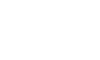How to calculate the power of the electric heater
Air electric heaters are becoming more and more widely used in industry. Many people are thinking about a problem. How to calculate the power of air heaters? Here, Kaitong Electric technicians will tell you.

To calculate the power level, you first need to know several parameters: flow rate, starting temperature, and temperature to be heated. Knowing these parameters can calculate the power of the air heater.

The calculation formula is: flow rate (kg/s)*1.29*1.009* (temperature required for heating-starting temperature)*1.3.

Among them, 1.29 is the air density, 1.009 is the specific heat of the air, the heating degree is required - the over temperature is the temperature rise, and the safety factor is 1.3.

For example, 4 cubic meters per minute, heated from 0 degrees to 10 degrees. This calculation: (4/60)*1.29*1.009*10*1.3=1.12kw. Ok, how do you calculate the power of the air heater?

Time :
How to calculate the power of the electric heaterNEWS